# Solving Circuits Solving Circuits Many circuits will have

• Slides: 10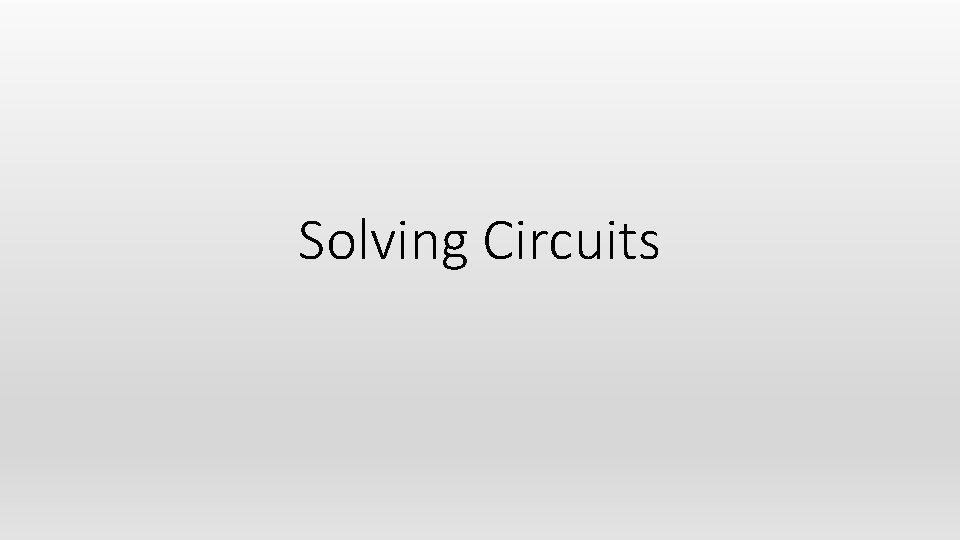Solving Circuits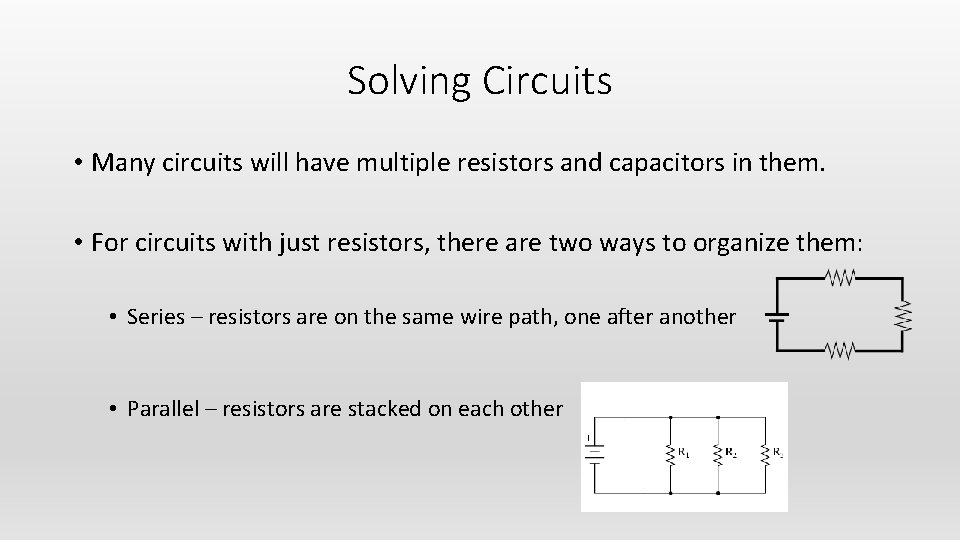Solving Circuits • Many circuits will have multiple resistors and capacitors in them. • For circuits with just resistors, there are two ways to organize them: • Series – resistors are on the same wire path, one after another • Parallel – resistors are stacked on each other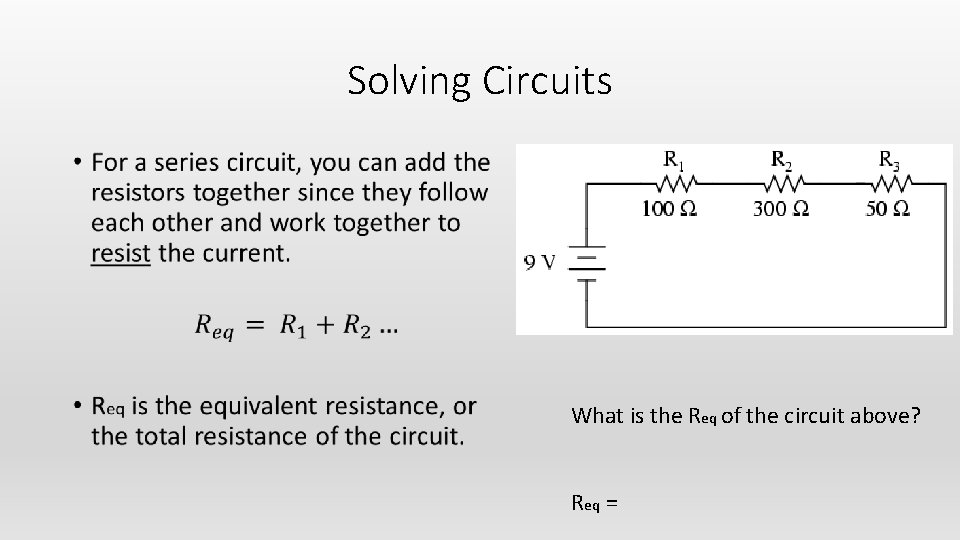Solving Circuits • What is the Req of the circuit above? Req =Solving Circuits • Electric current has but a single pathway through the circuit. This means that the current passing through each electric device is the same. • The current in the entire circuit is equal to the voltage supplied by the source divided by the total resistance of the circuit. (Ohm’s law) • For each resistor, there is a voltage drop as current goes through each resistor, which can be calculated with Ohm’s law. The voltage goes to 0 when the current reaches the other side of the battery.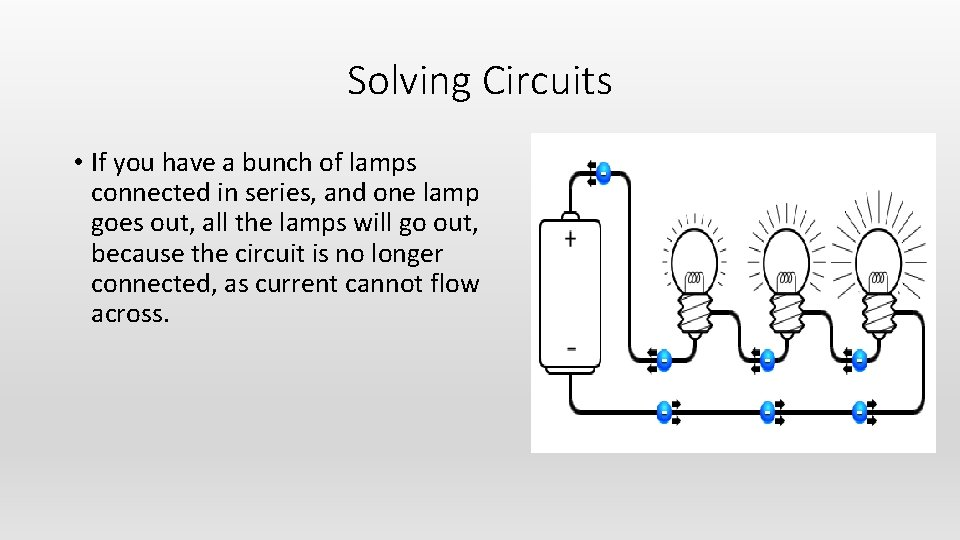Solving Circuits • If you have a bunch of lamps connected in series, and one lamp goes out, all the lamps will go out, because the circuit is no longer connected, as current cannot flow across.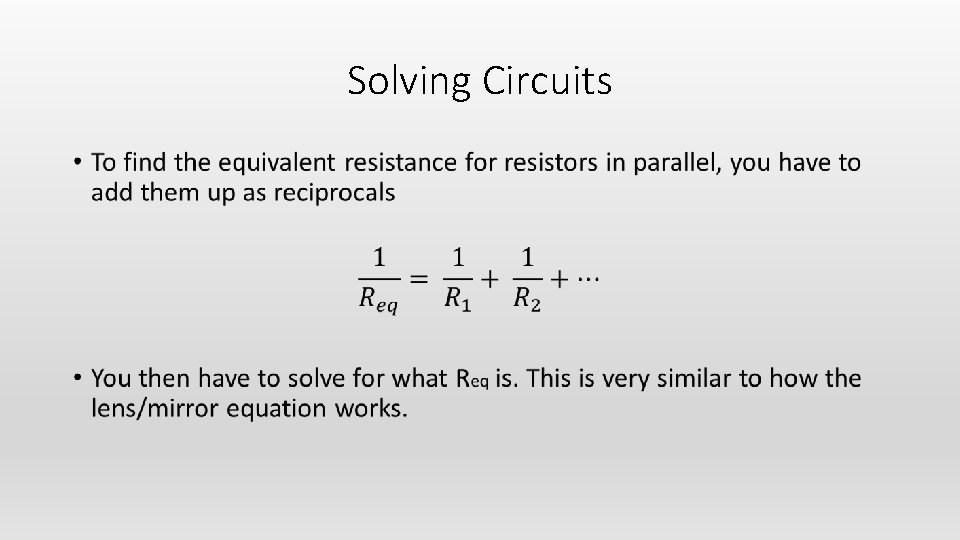Solving Circuits •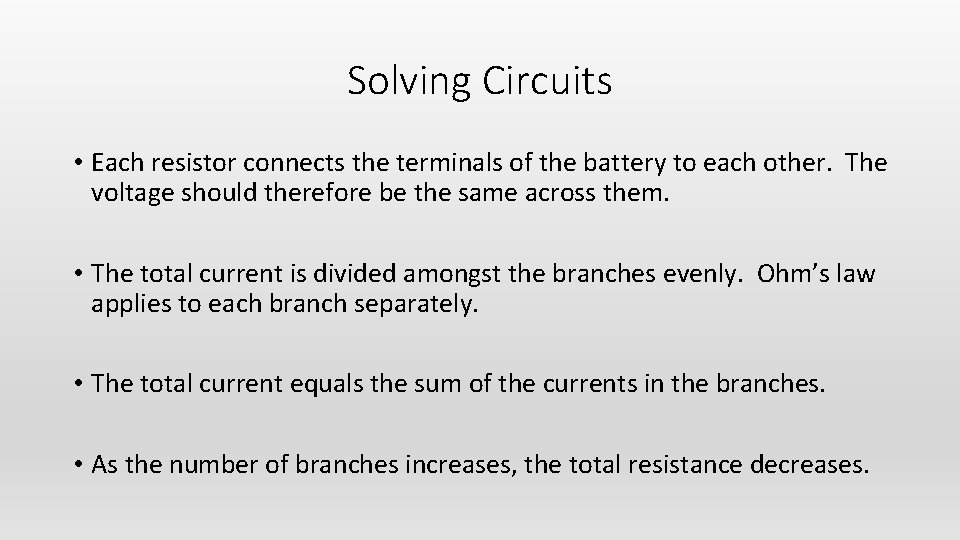Solving Circuits • Each resistor connects the terminals of the battery to each other. The voltage should therefore be the same across them. • The total current is divided amongst the branches evenly. Ohm’s law applies to each branch separately. • The total current equals the sum of the currents in the branches. • As the number of branches increases, the total resistance decreases.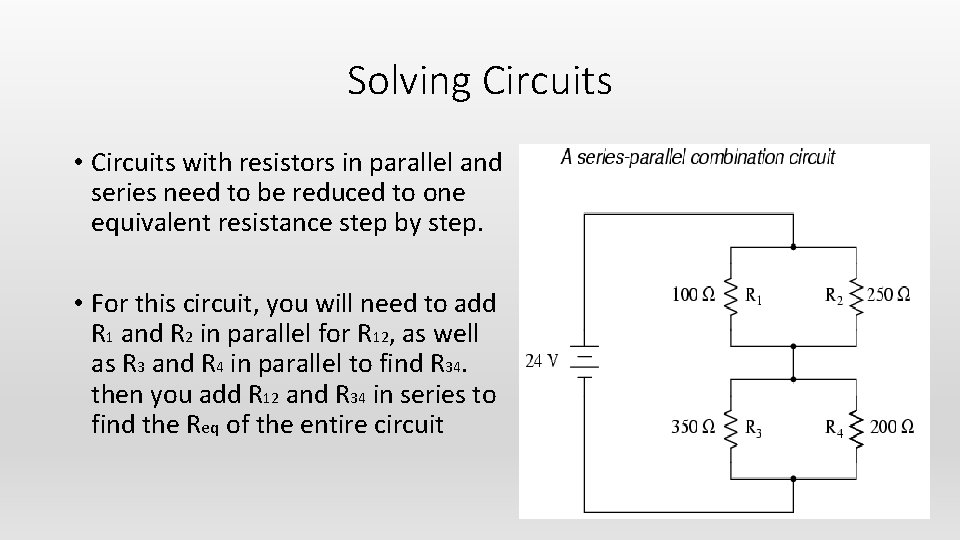Solving Circuits • Circuits with resistors in parallel and series need to be reduced to one equivalent resistance step by step. • For this circuit, you will need to add R 1 and R 2 in parallel for R 12, as well as R 3 and R 4 in parallel to find R 34. then you add R 12 and R 34 in series to find the Req of the entire circuit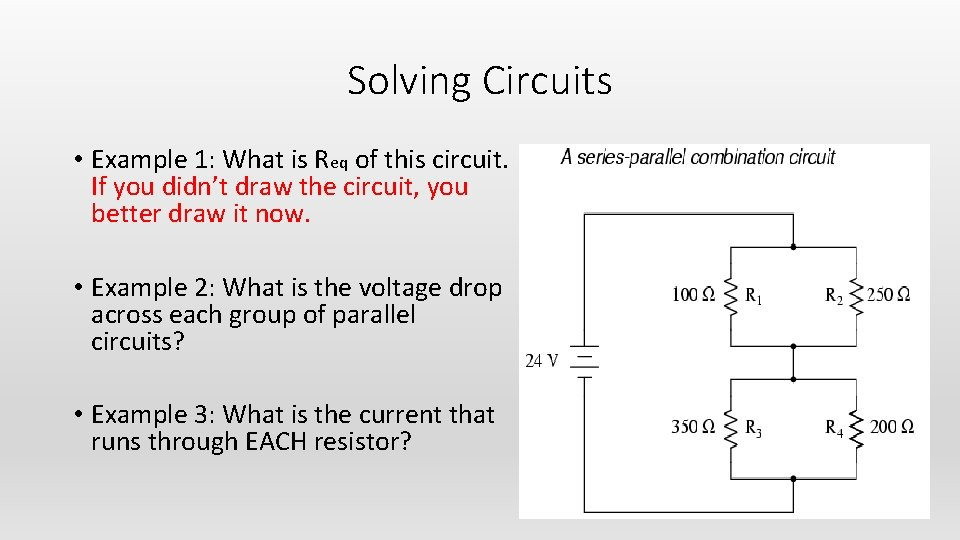Solving Circuits • Example 1: What is Req of this circuit. If you didn’t draw the circuit, you better draw it now. • Example 2: What is the voltage drop across each group of parallel circuits? • Example 3: What is the current that runs through EACH resistor?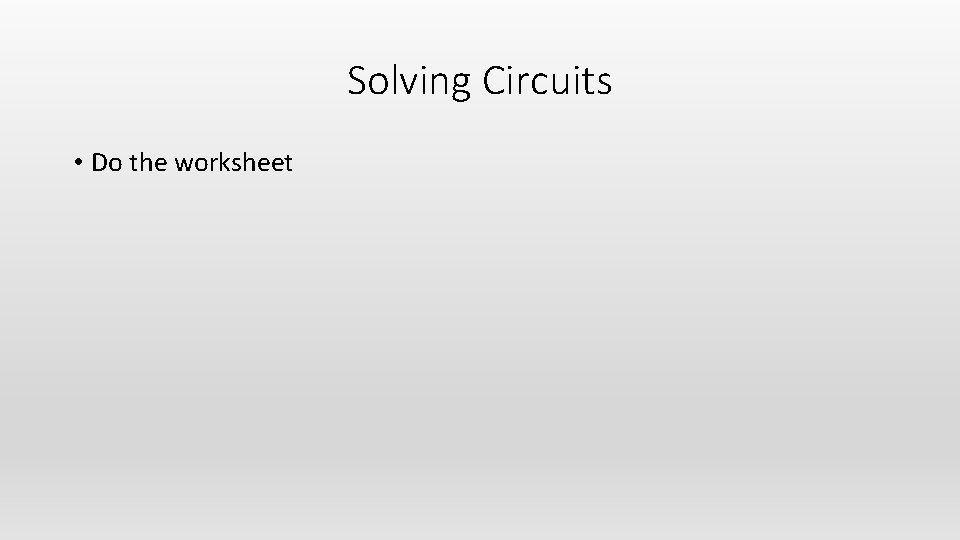Solving Circuits • Do the worksheet